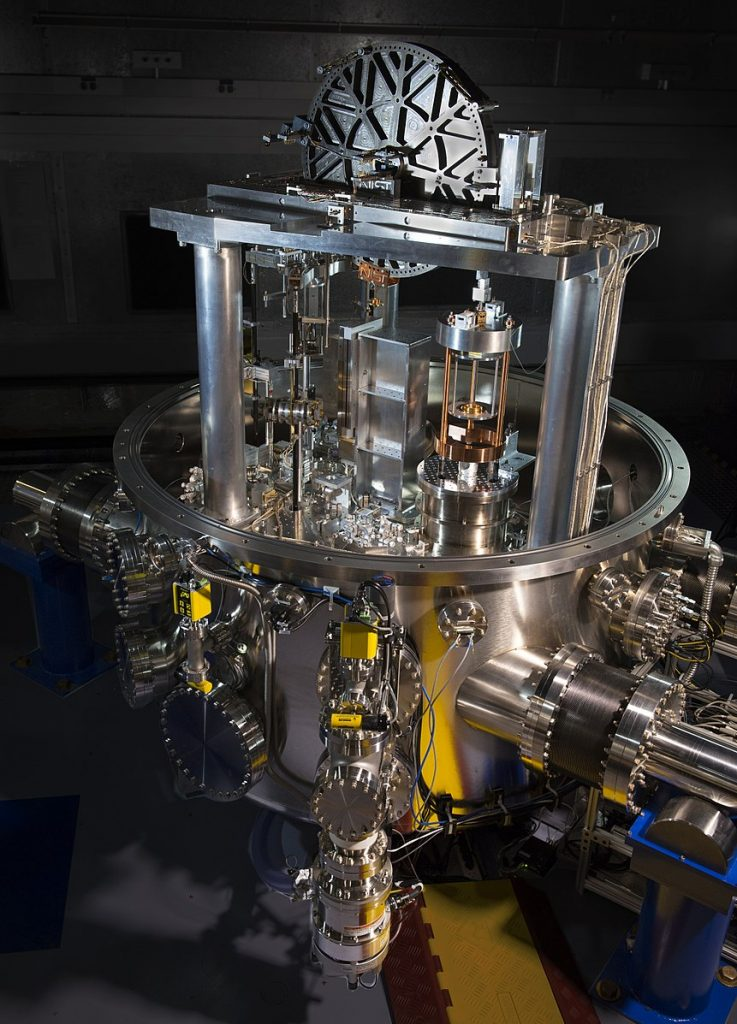# Electronic Kilogram

The kilogram is the only metric unit still defined by an artifact. The International Prototype Kilogram, IPK or “Le Grand K”, is a golf-ball-sized platinum-iridium cylinder in a vault outside Paris. This year I expect the General Conference on Weights and Measures to replace the IKP by an electronic realization that balances gravitational and electrical power.

The Kelvin or Ampere balance suspends a horizontal wire loop of mass $m$, length $\ell$, and current $I$, by a radial magnetic field $B$. Integrate the magnetic force $\overrightharpoon{F}=q\overrightharpoon{v}\times\overrightharpoon{B}$ around the loop to find the force balance

$$m g = F = \left| \oint d \overrightharpoon{F} \right| = \left| \oint dq \,\overrightharpoon{v} \times \overrightharpoon{B}\right| = \left| \oint I d\overrightharpoon{\ell} \times \overrightharpoon{B}\right| =I \ell B$$

and solve for $m$. Unfortunately, $\ell$ and $B$ are difficult to measure accurately.

In 1975, Bryan Kibble proposed the calibration step of moving the current-less wire loop vertically at speed $v$. Integrate the force per charge $\overrightharpoon{F}/q=\overrightharpoon{v}\times\overrightharpoon{B}$  around the loop to find the induced voltage

$$V = \oint \overrightharpoon{E} \cdot d\overrightharpoon{\ell} = \oint \frac{\overrightharpoon{F}}{q}\cdot d\overrightharpoon{\ell} = \oint \overrightharpoon{v} \times \overrightharpoon{B} \cdot d\overrightharpoon{\ell} = v B \ell.$$

Eliminate $\ell$ and $B$ from the force and voltage expressions to find the virtual power

$$P = V I = v B L I = m g v$$

in Watts, and again solve for $m$. Accurately measure voltage $V$ by comparing to the superconducting Josephson-effect voltage

$$V =\frac{n_J f}{K_J},$$

where $K_J = 2 e / h = 0.48~\text{THz} / \text{mV}$ is the Josephson constant$n_J$ is the number of Josephson junctions, and $f$ is their microwave frequency. Convert current $I = V_R / R$ to voltage and resistance by Ohm’s law. Accurately measure resistance $R$ by comparing to the quantum Hall-effect resistance

$$R =\frac{R_K}{n_L},$$

where $R_K = h /e^2 = 26~\text{k}\Omega$ is the von Klitzing constant, and $n_L$ is the number of filled Landau levels. Accurately measure velocity $v$ and acceleration $g$ using interferometers.

Hence the mass

$$m=\frac{VI}{gv}=VV_R\frac{1}{R}\frac{1}{gv}=n_J f\left(\frac{h}{2e}\right) n_J f_R\left(\frac{h}{2e}\right) n_L\left(\frac{e^2}{h}\right)\frac{1}{gv}=\frac{n_L n_J^2 f f_R h}{4gv}\propto h,$$

where $h=0.66~\text{zJ} / \text{THz}$ is the Planck constant. The Kibble or Watt balance thus defines mass in terms of the rate of change of a photon’s energy with its frequency.The NIST-4 Kibble balance has measured the Planck constant to 13 parts per billion and is thus accurate enough to help redefine the kilogram. Credit: Jennifer Lauren Lee.